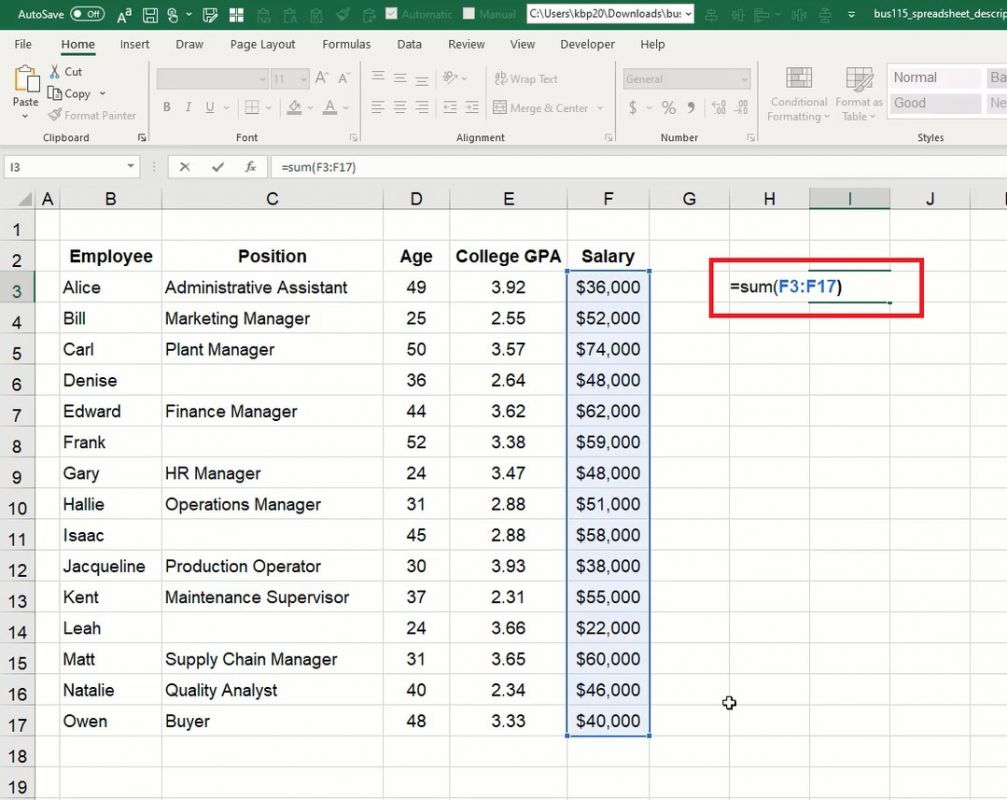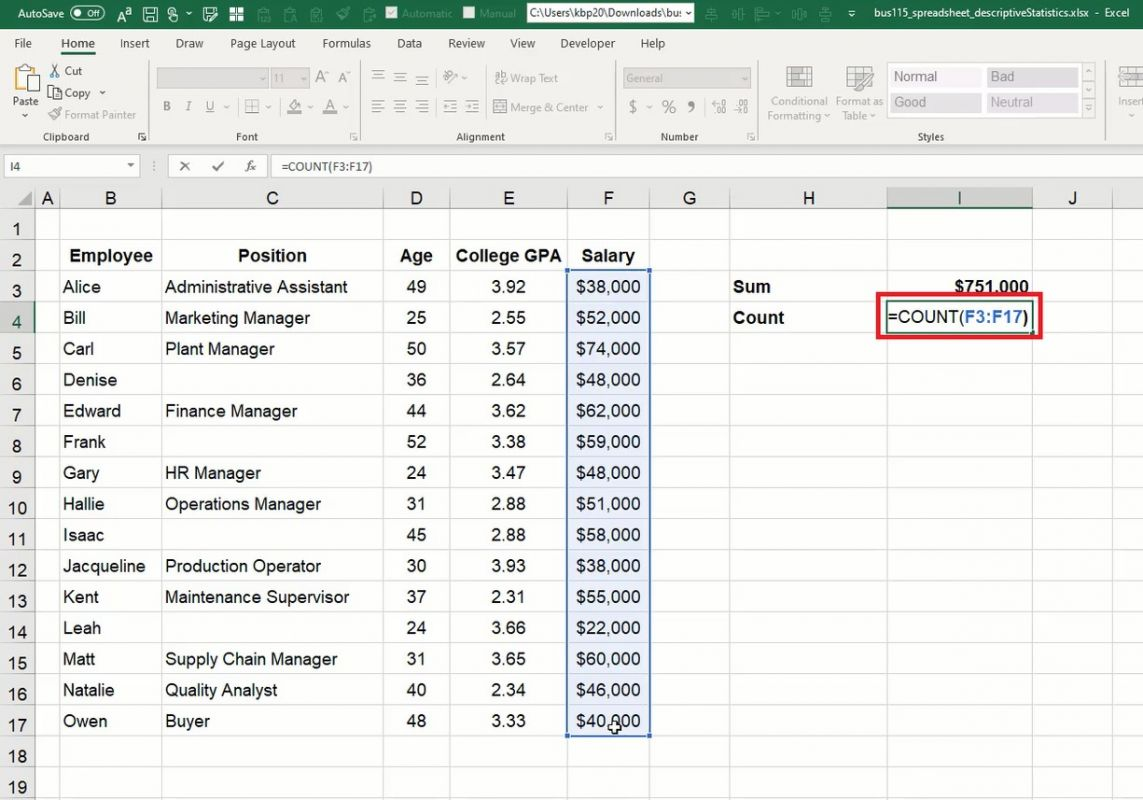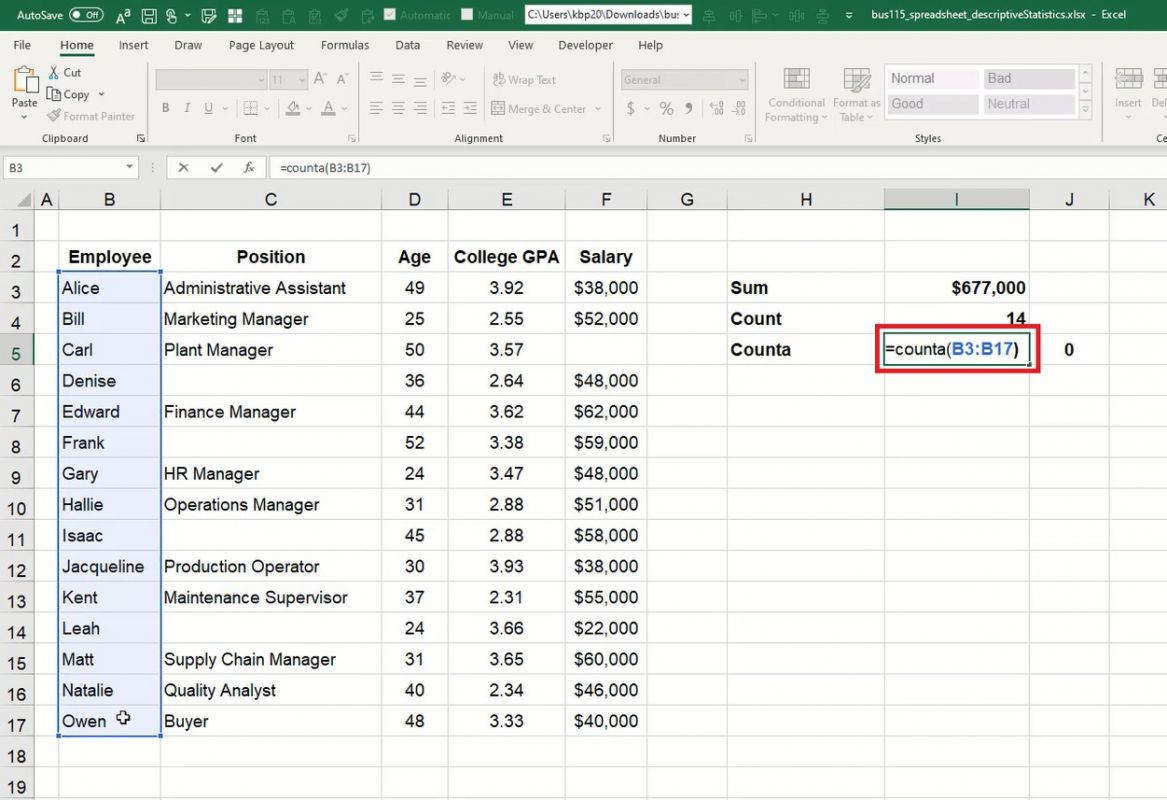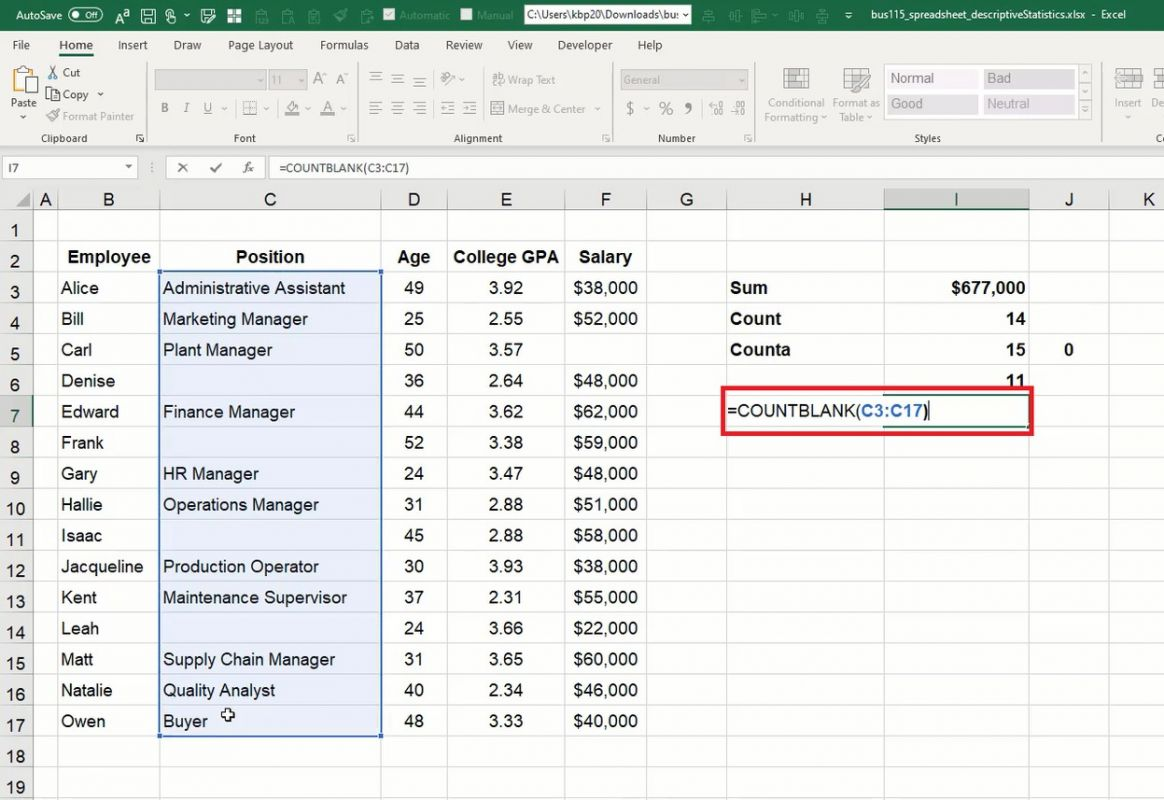# Sum and Count

In this chapter, you will learn how to summarize data with the sum and count functions. We have a table of data of some employees at a business. We have the name of the employee, their position, age, college GPA, and current salary. (See Figure 6.1)

Use this workbook for the chapter.

Note: This is the same workbook used in the Managing Worksheets chapter.

## Sum Function

Let’s look at the salary column. Let’s suppose that we want to calculate the sum total of all the employees’ salaries. We can do this with the Sum function.

1. Select an empty cell and type an equals symbol ( = ) to start the formula.
2. Type sum and an open (left) parentheses ( ( ).
3. Select all of the data to sum (F3 to F17 in Figure 6.2).
4. Type a close (right) parenthesis ( ) ) to close the formula data reference.
5. Press Enter to complete the cell’s formula (=sum(F3:F17)).
1. The total comes to $749,000. 2. If one of the salaries changes, then the sum total will automatically reflect that change. For example, if the F3 cell’s data changes from$36,000 to $38,000, the sum total will reflect the change:$751,000.Figure 6.2

## Count Function

Let’s suppose that we want to count the number of salaries in the list. We can do it with the Count function. The Count function counts numerical data only; it will not recognize text.

1. Select an empty cell and type an equals symbol ( = ) to start the formula.
2. Type count and an open (left) parenthesis ( ( ).
3. Select all of the data to count (F3 to F17 in Figure 6.3).
4. Type a close (right) parenthesis ( ) ) to close the formula data reference.
5. Press Enter to complete the cell’s formula (=count(F3:F17)).
1. The total count of salaries in Figure 6.3 is 15.
2. If one or more of the counted data is deleted, the count will recognize that change. For example, if the salary data for Carl in cell F5 is deleted, the total count will change from 15 to 14.Figure 6.3

## CountA Function

Another count function is the CountA Function. This function works the same as the Count function, except it is used to count non-numerical data—textual data such as names or positions.

1. Select an empty cell and type an equals symbol ( = ) to start the formula.
2. Type counta and an open (left) parenthesis ( ( ).
3. Select all of the data to count (B3 to B17 in Figure 6.4).
4. Type a close (right) parenthesis ( ) ) to close the formula data reference.
5. Press Enter to complete the cell’s formula (=counta(B3:B17)).
1. The total count of employees is 15.
2. If another CountA is inserted for the Positions column, the total count of positions comes to 11. The difference between the results reveals 4 employees do not have positions.Figure 6.5

## CountBlank Function

The CountBlank function can tell us the number of blank cells in a data set. In this case, we can verify the number of employees without positions from the CountA function example.

1. Select an empty cell and type an equals symbol ( = ) to start the formula.
2. Type countblank and an open (left) parenthesis ( ( ).
3. Select all of the data to count (C3 to C17 in Figure 6.5).
4. Type a close (right) parenthesis ( ) ) to close the formula data reference.
5. Press Enter to complete the cell’s formula (=countblank(C3:C17)).
1. The total count of blank cells is 4 in the Positions data set.Figure 6.5

### End-of-Chapter Survey

: How would you rate the overall quality of this chapter?
1. Very Low Quality
2. Low Quality
3. Moderate Quality
4. High Quality
5. Very High Quality
Comments will be automatically submitted when you navigate away from the page.